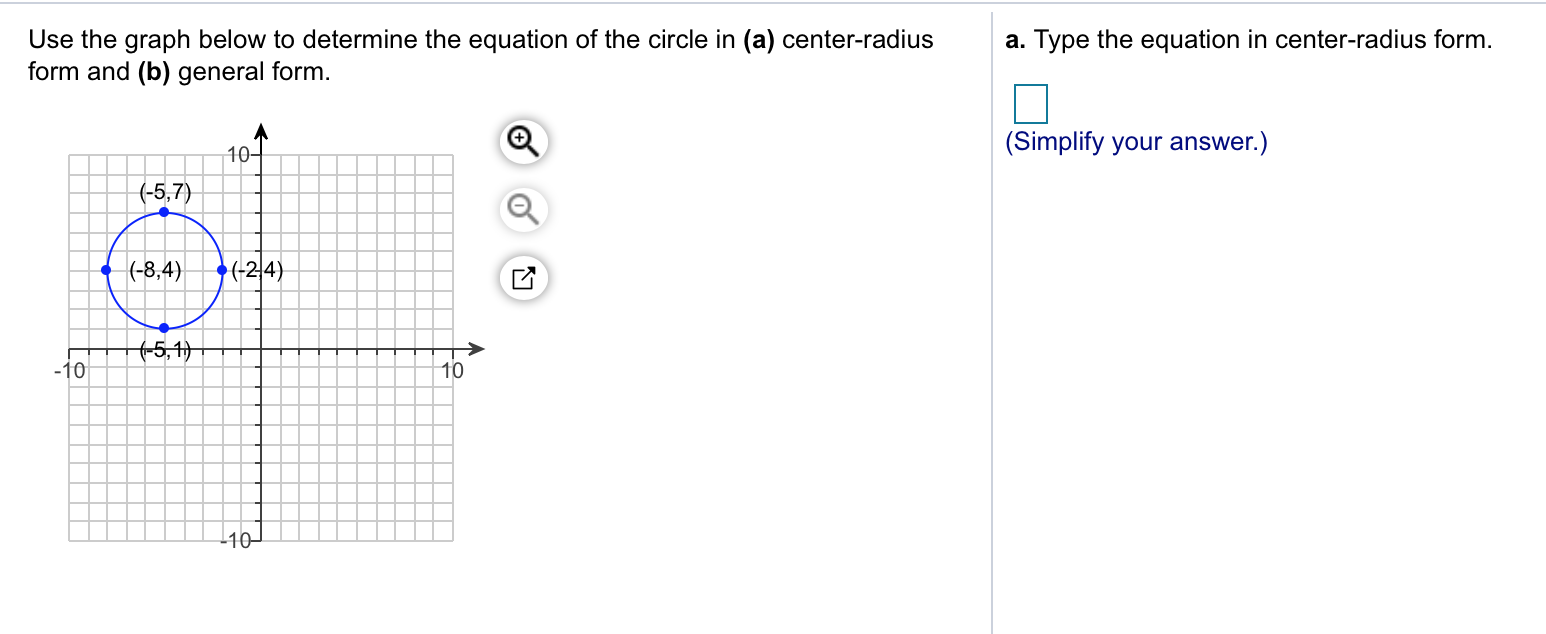# Use the graph below to determine the equation of the circle in (a) center-radiusa. Type the equation in center-radius formform and (b) general form(Simplify your answer.)10-5.7(-8,4) 24)1010

Question
25 viewshelp_outlineImage TranscriptioncloseUse the graph below to determine the equation of the circle in (a) center-radiusa. Type the equation in center-radius form form and (b) general form (Simplify your answer.) 10 -5.7 (-8,4) 24) 10 10 fullscreen
check_circle

Step 1

Formula used:

Equation of circle whose centre (h, k) and radius r is

(x - h)2 + (y - k)2 = r2

Step 2

According to the given graph the centre is the mid point of (-8, 4) and (-2, 4) is

(h, k) = [(-8-2)/2, (4+4)/2]

(h, k) = (-5, 4)

And diameter is distance between ((-8, 4) and (2, 4) is

Step 3

a) So equation of circle whose centre is (-5 , 4) and radius 3 ...

### Want to see the full answer?

See Solution

#### Want to see this answer and more?

Solutions are written by subject experts who are available 24/7. Questions are typically answered within 1 hour.*

See Solution
*Response times may vary by subject and question.
Tagged in

### Other